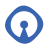#Open Source Glasgow

### Site Tools

coding:the_quiz_app_v2

# The Quiz App Version 2

At this point, you should have a basic, working quiz that is pulling questions from a database. If not, take a look at The Quiz App to get started.

This is what your code should look like before we get started, make sure you understand each line (and that it works). Look at the Troubleshooting section below if you run into problems.

```<?php
error_reporting(E_ALL);
ini_set("display_errors", 1);

require("questions_from_db.php");

\$size_of_question_list = sizeof(\$questions);

// check if we are in the middle of answering a question (if we are, then
// the random_number will already be set), otherwise, choose a number

if(empty(\$_REQUEST['random_number'])) {
\$random_number = rand(1, \$size_of_question_list);  // there is a bug here (we fixed it with the -1)
} else {
\$random_number = \$_REQUEST['random_number'];
}

?>

<h1>Quiz</h1>

<?php

\$question_id = \$_REQUEST['random_number'];

echo "Correct :)";
} else {
echo "Wrong :(";
}

}

?>

<p><strong>Question: </strong><?= \$questions[\$random_number]['question']; ?></p>
<form method='post' action='quiz.php'>
<input type='hidden' name='random_number' value='<?= \$random_number; ?>' />
</form>

<p><a href='quiz.php'>Another random question</a></p>```

## Sessions

You can store information in a session, which can be accessed on each page load. We'll use a session now to track which questions have already been answered, and we can record the name of person taking the quiz for a future high score table.

You can access what is in a session array in the same way you can see what is in a request array:

`print_r(\$_SESSION);`

Let's understand sessions first, separate from the quiz code. Create a new test page and call it `testsession.php`:

```<?php
session_start();
?>
<html>
<body>
<?php
if(!empty(\$_REQUEST)) {
print_r(\$_REQUEST);
}

if(!empty(\$_REQUEST['visitor_name'])) {
\$_SESSION['visitor_name'] = \$_REQUEST['visitor_name'];
}

if(!empty(\$_SESSION['visitor_name'])) {
echo "<p>Welcome ".htmlentities(\$_SESSION['visitor_name'])."</p>";
}

?>
<form method='post' action=''>
<input type='text' name='visitor_fullname' />
</form>
<?php

?>```

The above code has an error in it. See if you can spot where. I'll talk people through it.

Some functions we'll need:

phpinfo();

## Troubleshooting

### I just get a blank white screen or HTTP ERROR 500

```error_reporting(E_ALL);
ini_set("display_errors", 1);```

Remember to also add to any other files you might be including [or require()'ing]

Make sure you are using the correct credentials in `questions_from_db.php`. If you are unsure, you can set them again by connecting to your mysql server on the terminal:

`sudo mysql -u root`

Then, inside the mysql client, create a user called ‘coding_username’, with the password ‘cheese’ and give it access to the newly created ‘coding’ database:

```grant all on coding.* to coding_username@localhost identified by 'cheese';
quit```

Notice we use 'coding_username' - make sure that is what is in your `questions_from_db.php` file.

# Quiz App with Session Management

```<?php
session_start();
error_reporting(E_ALL);
ini_set("display_errors", 1);
?>
<h1>Quiz</h1>
<?php

if(!empty(\$_REQUEST['logout'])) {
session_destroy();
\$_SESSION = null;
}

if(!empty(\$_REQUEST['player_name'])) {
\$_SESSION['player_name'] = \$_REQUEST['player_name'];
}

if(empty(\$_SESSION['player_name'])) {
?>
<p>Welcome to the quiz, enter a name to begin:</p>
<form method='post' action=''>
<input type='text' name='player_name' placeholder='Your name' />
</form>
<?php
exit();
}

\$player_name = \$_SESSION['player_name'];

require("questions_from_db.php");
\$number_of_questions = sizeof(\$questions);

\$question_id = \$_REQUEST['random_number'];

echo "Correct :)";
\$_SESSION[\$player_name]['correct'][\$question_id] = \$question_id;
} else {
echo "Wrong :(";
\$_SESSION[\$player_name]['wrong'][\$question_id] = \$question_id;
}

}

if(!empty(\$_SESSION[\$player_name])) {
if(!empty(\$_SESSION[\$player_name]['correct']))
if(!empty(\$_SESSION[\$player_name]['wrong']))
if(!empty(\$_SESSION[\$player_name]['correct'])) {
foreach(\$_SESSION[\$player_name]['correct'] as \$question_id) {
unset(\$questions[\$question_id]);
}
}
if(!empty(\$_SESSION[\$player_name]['wrong'])) {
foreach(\$_SESSION[\$player_name]['wrong'] as \$question_id) {
unset(\$questions[\$question_id]);
}

}
}

?>

<h2>Welcome <?= htmlentities(\$_SESSION['player_name']); ?></h2>
<a href='quiz.php?logout=1'>Logout</a>
<?php

if(\$available_questions > 0) {
\$random_question_id = array_rand(\$questions);
?>

<p><strong>Question: </strong><?= \$questions[\$random_question_id]['question']; ?></p>
<form method='post' action='quiz.php'>
<input type='hidden' name='random_number' value='<?= \$random_question_id; ?>' />
</form>

<p><a href='quiz.php'>Another random question</a></p>
<?php
} else {
?>
<?php
if(empty(\$_SESSION[\$player_name]['score_saved'])) {
\$sql = "insert into high_scores set name=?, correct=?, wrong=?, created_on=now()";
\$insert_stmt = \$db_connection->prepare(\$sql);
echo "Highscore saved!";
} else {
echo "Error saving highscore";
echo print_r(\$insert_stmt->errorInfo());
}
} else {